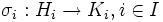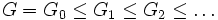# Every torsion-free group is a subgroup of a torsion-free group with two conjugacy classes

This article gives the statement, and possibly proof, of an embeddability theorem: a result that states that any group of a certain kind can be embedded in a group of a more restricted kind.
View a complete list of embeddability theorems

## Statement

Suppose$G$ is a torsion-free group. Then, there exists a torsion-free group with two conjugacy classes$L$ that contains$G$. Further, if$G$ is countable, we can choose$L$ to be countable.

## Facts used

1. Isomorphic iff potentially conjugate: Suppose$\sigma_i:H_i \to K_i, i \in I$ is a collection of isomorphisms between subgroups of$G$. Then, there exists a group$G_1$ containing$G$, with elements$g_i \in G_1$, such that conjugation by$g_i$, restricted to$H_i$, is equal to the isomorphism$\sigma_i$. Moreover, if$G$ is torsion-free, we can choose$G_1$ to be torsion-free.

## Proof

Given: A torsion-free group$G$.

To prove: There exists a torsion-free group$L$ containing$G$ such that$L$ has two conjugacy classes.

Proof:

1. There exists a torsion-free group$G_1$ containing$G$ such that any two non-identity elements of$G$ are conjugate in$G_1$: Let$I$ be the set of all pairs of non-identity elements in$G$, and$\sigma_i:H_i \to K_i$ be the isomorphism between the cyclic subgroups generated by the first element of the pair and the cyclic subgroup generated by the second element of the pair (sending the generator to the generator). Note that such an isomorphism exists because all non-identity elements have the same order (infinite). By fact (1), there exists a group$G_1$ satisfying the required conditions.
2. There exists a chain$G = G_0 \le G_1 \le G_2 \le \dots$ where any two non-identity elements of$G_{i-1}$ are conjugate in$G_i$: This follows by repeating the previous step.
3. Define$L$ as the ascending union (or direct limit) of the$G_i$s. Then, any two non-identity elements of$L$ are conjugate in$L$.